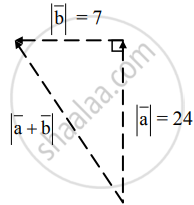# The vector aa¯ is directed due north and |a| = 24. The vector bb¯ is directed due west and b|b¯|=7. Find ab|a¯+b¯|. - Mathematics and Statistics

Sum

The vector bar"a" is directed due north and |bar"a"| = 24. The vector bar"b" is directed due west and |bar"b"| = 7. Find |bar"a" + bar"b"|.

#### SolutionLet bar"AB" = bar"a", bar"BC" = bar"b"

Then bar"AC" = bar"AB" + bar"BC" = bar"a" + bar"b"

Given: |bar"a"| = |bar"AB"|

= l("AB")

= 24

and

|bar"b"| = |bar"BC"|

= l("BC")

= 7

∵ ∠ABC = 90°

∴ ["l"("AC")]^2 = ["l"("AB")]^2 + ["l"(BC)]^2

= (24)^2 + (7)^2

= 625

∴ |l(AC)| = 25

∴ |bar"AC"| = 25

∴ |bar"a" + bar"b"| = |bar"AC"| = 25

Concept: Vectors and Their Types
Is there an error in this question or solution?